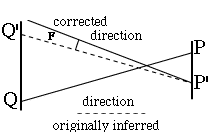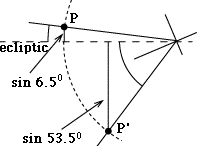# #12e   Deriving the Astronomical UnitIndex9c. Copernicus
to Galileo10. Kepler's Laws

Kepler's Laws
(For teachers)10a. Scale of Solar Sys.11. Graphs & Ellipses11a. Ellipses
and First Law12. Second Law12a. More on 2nd Law12b. Orbital Motion12c. Venus transit (1)12d. Venus transit (2)12e. Venus transit (3)

Newtonian Mechanics13. Free Fall14. Vectors15. Energy16. Newton's Laws17. Mass
Some of the numbers in eq.(8)

D = (R–r) [sin2 θ / cosθ] ΔT / T                 (8)

can be prepared beforehand. As already shown, (R–r) = 15.25' (minutes of arc), and with θ = 46.62° , sin2 θ/cosθ = 0.76124. For the two observing stations chosen here, with (hour:min:sec) given in universal time (Greenwich time), one gets from (no longer posted)
http://sunearth.gsfc.nasa.gov/eclipse/transit/TV2004.html#city

 Station 2nd contact 3rd contact T Durban   in seconds 5:35:52 20152 11:10:07 40207 20055 Cairo   in seconds 5:39:09 20349 11:04:35 39875 19526

Average T = 19.790.5 sec

Difference ΔT = 529 sec

ΔT/T = 0.02673

When the Venus transit method was first tried in 1769, precise observations of ΔT were foiled by an unexpected phenomenon. As the dark disk of Venus approached the edge ("limb") of the solar disk, a dark bridge seemed to form between it and the darkness beyond the disk, making it difficult to identify the moments of second and third contact. The cause of this "black drop effect" is still being debated, but it may be related to the steep decrease in the Sun's brightness near the Sun's limb. Such "limb darkening" (also evident in the Sun's image used in part 1 of this calculation) occurs because light reaching us from near the visible edge is by necessity emitted at a very shallow angle. It therefore has to travel through a great thickness of the cooler layers of the Sun (above the light-emitting photosphere but still below the chromosphere and corona), and part of it is absorbed again. Putting all the above in (8), where D (like R–r) is expected to be given in minutes of arc,

D = (15.25)(0.76124)(0.02673) = 0.310306'

or just 1/3 the width of the disk of Venus in front of the Sun.

We will assume that the transit paths across the Sun are parallel to the ecliptic, and we will only use the projection of PP' perpendicular to the ecliptic.

## The Earth's Orbit

We now need to introduce distances in the solar system as measured in astronomical units. The mean Sun-Earth distance is 1 AU, but it varies slightly because of the eccentricity of the Earth's orbit, e = 0.01673. (This value might in principle be deduced from the unequality of seasons, discussed in section #12a on Kepler's 2nd law, but the calculation is not a simple one.) The distance to any planet moving in a Kepler ellipse, in polar coordinates (r, θ), is given by

r = a(1-e2)/(1 + e cosθ)                 (9)

(note this use of the symbol "r" differs from the earlier one). The (smallest, largest) distances occur when θ= (0°,180°) and cosθ = (1, –1). Given the identity (1–e2) = (1–e)(1+e) those distances become r(1–e), r(1+e), or for the Sun-Earth distance in AU, just 1–e and 1+e.

Earth is closest to the Sun (=at perihelion) around January 4, so its greatest distance (at aphelion) should be in early July. The transit of June 8, 2004, was close to the latter, so as a guess let's put the distance at 1.015 AU (considering it is 1.01673 at aphelion, where it has a maximum and varies slowly, and 1 AU at spring equinox, near March 21).

But what is the distance of Venus in AU? Here one can use Kepler's 3rd law, by which the square of the orbital period T is proportional to the cube of the mean distance a (the semi-major axis). The period of Venus is 0.616 years, hence its mean distance from the Sun is 0.723 AU.

At transit time Venus is between us and the Sun, and if both orbits were circular, this would put Earth at 0.277 AU from Venus. In the absence of more information, a circular orbit will be assumed for Venus (actually, a very good approximation). However, since Earth is near aphelion, at an assumed distance of 1.015 AU, we need add 0.015 AU to the Earth-Venus distance, and get 0.292 AU.

## The Shift in the Sun's Apparent PositionFig. 5   The Sun's center viewed from P and from P'

Next a more subtle matter. The observation sites P and P' are some distance apart and as calculated, they see Venus at slightly different locations in the sky. However, The Sun too is at a finite distance from us, and the location of its edge--which serves as reference--also has slightly different positions in the sky, when seen from P and from P'.

Take the Sun's center O. If the Sun were transparent and we could see the stars behind it, viewing it from P and from P' would show slightly different backgrounds, and the two directions would form a small angle F (see drawing). The sky coordinates of any other point on the Sun (e.g. a small sunspot) would also have this difference when observed from P or P'. When comparing the shift D of the track of Venus, we need to add the shift F of our reference points (Figure 6).

We now denote by x the number of kilometers in one AU: that is the number we wish to derive! The long narrow triangle PP'O may be viewed as a sliver cut (like a pie section) from a circle with O at the center. The entire circle contains 360×60 = 21600 minutes of arc, and the ratio between F and that number is essentially the same as the ratio between the distance PP' (within a tiny error because PP' is straight) and the whole length of the circle, which is 2πx, with 2π=6.2832 to an accuracy of 4 figures past the decimal. Expressed in numbers

F / 21600 = PP'/(6.2832 (1.015 x))                 (10)

The angle F is very small, because the Sun is very far. Nevertheless, it cannot be ignored, because the angle D with which we are working is also extremely small.Fig. 6   Correcting the viewing angle for the Sun's finite distance

Let us now choose some instant during the transit, when P sees Venus at point Q (on line AB) and P' sees Venus at point Q' (on line A'B'). For this calculation, the sky-positions seen from P will serve as our "reference system," and "up" and "down" here will refer to the directions in the drawing. To get the "standard sky directions" of any point on the Sun seen from P' --including any point of A'B', and in particular Q'--its position needs to be "lifted upwards" by an angle F. (Figure 6)

## The Corrected Parallax Angle D'Fig. 7   Geometry of the corrected Venus parallax

To obtain the "sky position" of A'B' and of Q' in the same celestial coordinates as AB and Q, we must (as noted before) "lift" it by an angle F towards the center of the Sun. It follows then that the angle PVP' (or QVQ', which equals it) is not D but

D' = D + F         (11)

Earlier, when Figure (1b), was discussed, that angle was referred to as "D", which (as is now realized) is not completely correct. In fact the angle PVP' was never measured, it was only inferred from the observed positions of Venus in front of the Sun. Actually PVP' equals D', not the originally inferred angle D, because not just Venus but also the Sun behind it was observed from two different points.

Now in Figure 7 we have a long narrow triangle whose side is the Earth-Venus distance, estimated as Rv=0.292AU . Since the angle D' is very small, PP' may be regarded as small part of a circle, giving very nearly

PP'/ 2πRv = D'/360°

In Figure 5 the same argument can be applied, except the angle is now F and the side of the triangle is the Earth-Sun distance, estimated as Rs=1.015AU. Hence (very nearly)

PP'/ 2πRs = F/360°

Dividing right by right, left by left

Rs / Rv   =   D' / F   =   1.015/0.292   =   3.476         (12a)

Meaning that at the time of the transit, the Sun is about three and a half times more distant than Venus. From (10)

x   =   (PP' / F) [21600/(6.2832. 1.015)]   =   3386.9 (PP' / F)         (12b)

Combining equations (11) and (12a),

D  =  D' – F   =   F [(D'/F) – 1]  =   2.476 F

The value of D was 0.310306 minutes of arc. From (12b)

x   =   3386.9 PP' [2.476/D]   =   3386.9 PP' [2.476/0.310306]

x   =   27024.8 PP'         (13)

(Thanks to Prof. Udo Backhaus of the Univ. of Essen for pointing out some shortcuts here.)

## Derivation of the AU

On the summer solstice, around June 21, the Earth's axis is tilted towards the Sun, making an angle of 23.5 degrees with the line perpendicular to the ecliptic. As a crude approximation, let us assume that was also the condition on June 8 (Figure 8a).

Both locations are about 30° east of Greenwich, making their local time two hours later. The transit therefore starts before 8 am and ends around 1 pm. At local noon that day, the radius from the Earth's center to P (Cairo) made an angle to the ecliptic (see drawing) of

30° – 23.5° = 6.5°

and the perpendicular distance of P to the ecliptic, in units of the Earth radius RE, was

(sin 6.5)(1 RE) = 0.11320 REFig. 8a   Geometry of driving PP'

30° + 23.5° = 53.5°

and the perpendicular distance of P' from the ecliptic was

(sin 53.5°)(1 RE) = 0.80386 REFig. 8b   Projection of PP' perpendicular to the ecliptic

The separation of the points in the direction perpendicular to the ecliptic, which we chose as PP' (disregarding shifts between the points in the Sun-Earth direction, much smaller than the distances to Venus and to the Sun) was

PP'  =  0.11320 + 0.80386 = 0.91706 RE

It may be shown that at other local times, with PP' shifted by the Earth's rotation, its value is about the same (the shift is nearly parallel to the plane of the ecliptic). With an earth radius of 6371 km we get, to an accuracy of 4 figures

PP' = (0.91706)(6371) = 5842.6 km

Substituting in (13),

x = 157.9 million km

The accepted value is about 149.59 million km (often rounded up to 150 million) so the above value is about 5% off. (I have not traced the source of the discrepancy; a 1-degree error in the value of θ also gives that much change.)

By the way, astronomers often present this information in an equivalent way as the "solar parallax." That is the angle F obtained in Figure 5, if the two observing points are separated by one Earth radius (1 RE) and 1 AU distant. The common value given for the solar parallax is 8.79" (seconds of arc, 1/60 of a minute of arc). You may use eq. (10) to derive it.

Next Stop--first section on Newtonian Mechanics:       #13. The Way Things Fall

Author and Curator:   Dr. David P. Stern
Mail to Dr.Stern:   stargaze("at" symbol)phy6.org .

Last updated: 7 April 2014